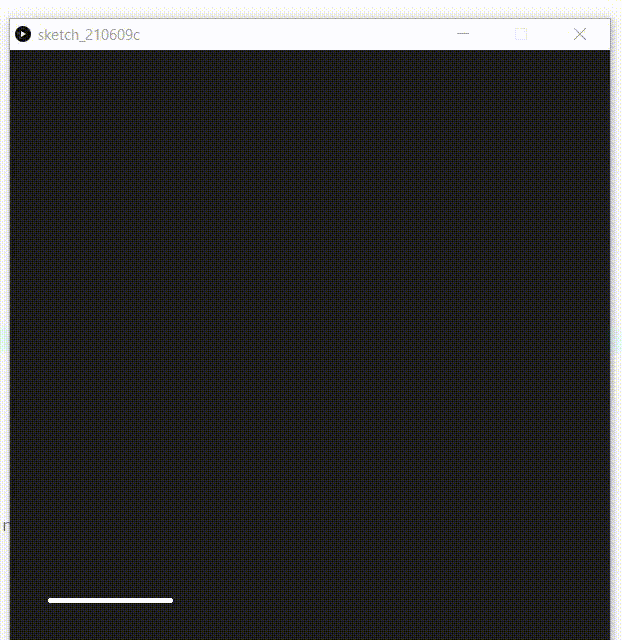Related Articles

# How to draw simple animation using p5.js ?

• Last Updated : 04 Oct, 2021

Animation is a method in which a collection of images is combined in a specific way and processed then they appear as moving images. Building animations make on-screen objects seem to be alive.

In this article, we will learn to make a simple animation of the house in p5.js by using lines, rectangles and ellipses for making the parts of the house.

Hey geek! The constant emerging technologies in the world of web development always keeps the excitement for this subject through the roof. But before you tackle the big projects, we suggest you start by learning the basics. Kickstart your web development journey by learning JS concepts with our JavaScript Course. Now at it's lowest price ever!

Approach:

• Make a list to store all the vertices of the house.
• Declare two variable iter and counter.
• Set the function setup() in which the size,colour and background of output window ,initiate the value of iter and counter as 1 and initialise the list of vertices.
• Set the function draw in which add stroke,  stroke weight.
• Make a if condition to check iter within bound if yes increase the counter by 0.05 and thake the ceil value of counter as iter , if no exit from the loo.
• Function to add vertices of house giving start and end point of line as addVertices().
• Now make functions to draw the parts of house:
• Make Function to draw vertical and horizontal  lines in house.
• Make Function to draw  square window
• Make Function to draw gate.
• Make Function to draw circular window.
• Make Function to draw chimney.
• After all this step now create a switch case to add all the parts of house step by step.

Below is the implementation of the above approach:

## Javascript

 `// List to store all the vertices``let vertices = [];` `// Variable declared``var` `iter;``var` `counter;` `// Function to set up output window``function` `setup() {` `    ``// Size of output window``    ``createCanvas(600, 600);` `    ``// Fill the color``    ``fill(31);` `    ``// Background of output window``    ``background(31);` `    ``// Put the value of variables as 1``    ``iter = 1;``    ``counter = 1;` `    ``// Initialize the list of vertices``    ``addVertices();``}` `// Set the draw function``function` `draw() {` `    ``stroke(255);``    ``strokeWeight(4);``    ``step();` `    ``// Condition to check within bound``    ``if` `(iter < 11) {` `        ``// Increase counter everytime``        ``counter += 0.05;` `        ``// Set the iter variable to the``        ``// floor value of counter``        ``iter = floor(counter);``    ``}``    ``else` `{` `        ``// If iter increases by 11 then``        ``// stop the loop``        ``noLoop();``    ``}``}` `// Function to add vertices of house giving``// start and end point of line``function` `addVertices() {``    ``vertices.push(``new` `p5.Vector(100, 300));``    ``vertices.push(``new` `p5.Vector(340, 300));``    ``vertices.push(``new` `p5.Vector(40, 380));``    ``vertices.push(``new` `p5.Vector(160, 380));``    ``vertices.push(``new` `p5.Vector(400, 380));``    ``vertices.push(``new` `p5.Vector(40, 550));``    ``vertices.push(``new` `p5.Vector(160, 550));``    ``vertices.push(``new` `p5.Vector(400, 550));``}` `// Function to draw lines in house``function` `drawLine(a, b) {``    ``line(vertices[a].x, vertices[a].y,``        ``vertices[b].x, vertices[b].y);``}` `// Function to draw gate``function` `addGate() {``    ``rectMode(CENTER);``    ``rect(100, 500, 70, 100);``}` `// Function  to draw window``function` `addWindow() {``    ``rect(280, 430, 40, 30);``}` `// Function to add  circular window``function` `addOculus() {``    ``ellipse(100, 340, 20, 20);``}` `// Function to add Chimney``function` `addChimney() {``    ``rect(320, 295, 16, 20);``    ``ellipse(320, 285, 16, 10);``}` `// Function to draw parts of``// house step by step``function` `step() {``    ``switch` `(iter) {``        ``case` `1:``            ``drawLine(5, 6);``            ``break``;``        ``case` `2:``            ``drawLine(6, 7);``            ``break``;``        ``case` `3:``            ``drawLine(2, 5);``            ``drawLine(3, 6);``            ``break``;``        ``case` `4:``            ``drawLine(4, 7);``            ``break``;``        ``case` `5:``            ``drawLine(2, 3);``            ``drawLine(3, 4);``            ``break``;``        ``case` `6:``            ``drawLine(0, 2);``            ``drawLine(0, 3);``            ``drawLine(1, 4);``            ``break``;``        ``case` `7:``            ``drawLine(0, 1);``            ``break``;``        ``case` `8:``            ``addGate();``            ``break``;``        ``case` `9:``            ``addWindow();``            ``break``;` `        ``case` `10:``            ``addOculus();``            ``break``;``        ``case` `11:``            ``addChimney();``            ``break``;``    ``}``}`

Output:My Personal Notes arrow_drop_up